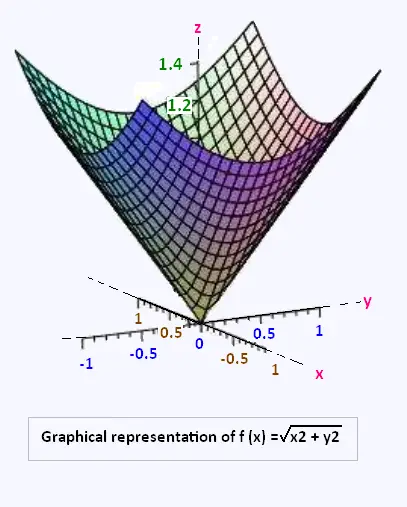###### Mathematics

functions of
several variables

functions of
several variables

Partial derivatives
Differential

Linear approximation

Error calculation

Extrema of a function

© The scientific sentence. 2010

Mathématiques 2chimie labs | Physics and Measurements | Probability & Statistics | Combinatorics - Probability | Chimie | Optics | contact |